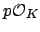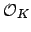## Ideals

```> R<x> := PolynomialRing(RationalField());
> K<a> := NumberField(x^3-2);
> O := Order([2*a]);
> O;
Transformation of Order over
Equation Order with defining polynomial x^3 - 2 over ZZ
Transformation Matrix:
[1 0 0]
[0 2 0]
[0 0 4]
> OK := MaximalOrder(K);
> Index(OK,O);
8
> Discriminant(O);
-6912
> Discriminant(OK);
-108
> 6912/108;
64    // perfect square...
> R<x> := PolynomialRing(RationalField());
> K<a> := NumberField(x^2-7);
> K<a> := NumberField(x^2-5);
> Discriminant(K);
20   // ????????? Yuck!
> OK := MaximalOrder(K);
> Discriminant(OK);
5    // better
> Discriminant(NumberField(x^2-20));
80
> I := 7*OK;
> I;
Principal Ideal of OK
Generator:
[7, 0]
> J := (OK!a)*OK;    // the ! computes the natural image of a in OK
> J;
Principal Ideal of OK
Generator:
[-1, 2]
> I*J;
Principal Ideal of OK
Generator:
[-7, 14]
> J*I;
Principal Ideal of OK
Generator:
[-7, 14]
> I+J;
Principal Ideal of OK
Generator:
[1, 0]
>
> Factorization(I);
[
<Principal Prime Ideal of OK
Generator:
[7, 0], 1>
]
> Factorization(3*OK);
[
<Principal Prime Ideal of OK
Generator:
[3, 0], 1>
]
> Factorization(5*OK);
[
<Prime Ideal of OK
Two element generators:
[5, 0]
[4, 2], 2>
]
> Factorization(11*OK);
[
<Prime Ideal of OK
Two element generators:
[11, 0]
[14, 2], 1>,
<Prime Ideal of OK
Two element generators:
[11, 0]
[17, 2], 1>
]
```
We can even work with fractional ideals in .
```> K<a> := NumberField(x^2-5);
> OK := MaximalOrder(K);
> I := 7*OK;
> J := (OK!a)*OK;
> M := I/J;
> M;
Fractional Principal Ideal of OK
Generator:
-7/5*OK.1 + 14/5*OK.2
> Factorization(M);
[
<Prime Ideal of OK
Two element generators:
[5, 0]
[4, 2], -1>,
<Principal Prime Ideal of OK
Generator:
[7, 0], 1>
]
```

In the next chapter, we will learn about discriminants and an algorithm for ``factoring primes'', that is writing an idealas a product of prime ideals of.

William Stein 2004-05-06